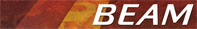NDVI Algorithm SpecificationAlgorithm Specification of the science processors of the MERIS/(A)ATSR Toolbox

### NDVI

The Normalized Difference Vegetation Index algorithms exploit the strength and the vitality of the vegetation on the earth's surface.

The spectral signatur of wholesome vegetation shows a abrupt rise of the reflection level at 0,7 µm, whereas land without vegetation, according to the type of surface, has a continuous linear course. So much more active the chlorophyll of the plants, so much bigger is the boost of the reflection level at the near infrared (0,78 - 1 µm). Beside the determination between of the vegetation and other objects it allows to detect the vitality of the vegetation.

The NDVI results from the following equation:

NDVI = (IR_factor * near_IR - red_factor * red) / (IR_factor * near_IR + red_factor * red)

In the area of red the incoming solar radiation won´t be extensively absorbed by the pigments of the mesophyll inside the folios, primarily by the chlorophyll. In the area of the near infrared in contrast the bigger part of the incoming radiation was reflected. The NDVI composes a measurement for the photosynthetic activity and is strongly in correlation with density and vitality of the vegetation. The normalizing reduces topographicaly and atmospharic effects and enables the simultaneous examination of a wide area.

Applied to the MERIS Level 1b TOA radiances, using the following bands and factors:

 As the near IR band: radiance_10 (753.75 nm) As the IR factor: 2.0 As the red band: radiance_6 (620.00 nm) As the red factor: 1.0

Leads to the following equation:

NDVI = (IR_factor * radiance_10 - red_factor * radiance_6) / (IR_factor * radiance_10 + red_factor * radiance_6)

### NDVI-Flags

Also the processor computes an additional flags band called 'ndvi_flags' with the following bit coding:

Bit PositionDescription
Bit 0The computed value for NDVI is NAN or is Infinite
Bit 1The computed value for NDVI is less than 0 (zero)
Bit 2The computed value for NDVI is gerater than 1 (one)